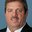Question-and-Answer Resource for the Building Energy Modeling Community
Get started with the Help page

# two-speed, multi-speed, variable-speed difference?Other than the number of speeds allowed, is there a difference between these three types of DX cooling? Do all work with AirLoopHVAC:UnitarySystem? If I model only two speeds, would all give the same results?

edit retag close merge delete

@Rohini I changed your tags to include the existing cooling-coil (singular per UH convention) and appended coil to the speed tags to make less vague.

Sort by » oldest newest most voted1) Other than the number of speeds allowed, is there a difference between these three types of DX cooling?

The models were developed at different times yet are fundamentally the same. All use bi-quadratic performance curves for capacity and EIR as a function of entering wet-bulb (indoor coil) and dry-bulb temperature (outdoor coil):

$Curve_{output}$ $= a + b * wb + c * wb^2 + d * edb + e * edb^2 + f * wb * edb$

and quadratic or cubic curves for performance as a function of flow fraction:

$Curve_{output}$ $= a * b * ff + c * ff^2$
$Curve_{output}$ $= a * b * ff + c * ff^2 * d x ff^3$

The two-speed model has 2 speeds, the multi-speed model has up to 4 speeds, and the variable-speed model has up to 10 speeds.

The two-speed model does have additional curves to model changes in SHR based on temperature and flow if the manufacturer has provided sufficient data to model this aspect of performance (otherwise leave these curves blank or set to 1).

Sensible Heat Ratio Function of Temperature Curve Name
Sensible Heat Ratio Function of Flow Fraction Curve Name


2) Do all work with AirLoopHVAC:UnitarySystem?

These coils are all allowed with the AirLoopHVAC:UnitarySystem. Remember that this object is new to EnergyPlus and there may be some programmatic differences (which should not exist) that were missed during coding. These issues are being resolved as they are discovered.

3) If I model only two speeds, would all give the same results?

Optimally yes. However, these models rely on different algorithms to calculate performance which may lead to slight differences in results.

more

Is it possible to find info on the different algorithms and the differences (may be the engineering reference manual?). Which one is using the most recent algorithm (the variable-speed?). By algorithm, are you referring to the DX coil model and how it calculates the sensible/latent loads or just the overall modelling for a unitary system?

Yes, the engineering reference shows the equations used for each model. The VS model is the most recent, but by algorithm I was referring to the subroutine called by each coil. These subroutines could use slightly difference approaches to calculating the results.

@rraustad I tried changing your equations to MathJax format because there was MarkDown collision with the *. It dispalyed correctly in the edit preview, but for some reason isn't here.